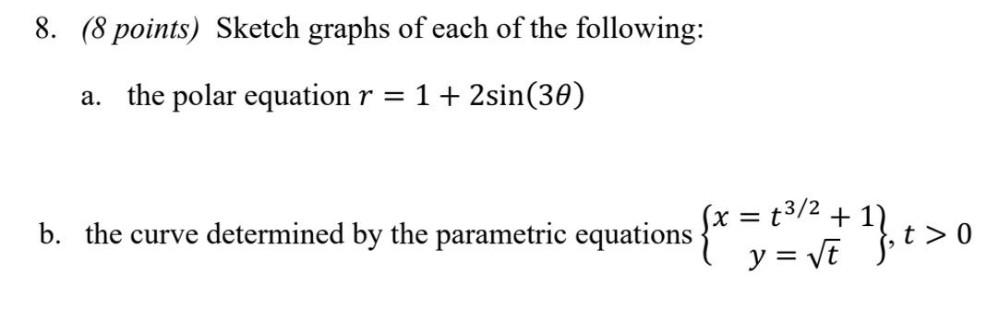Question:

# Sketch graphs of each of the following: (a) the polar

Last updated: 7/31/2022Sketch graphs of each of the following: (a) the polar equation r = 1 + 2sin(3θ) (b) the curve determined by the parametric equations { x = t³/² + 1 } { y = √t }, t > 0